QlikView Documents

QlikView documentation and resources.

Announcements
QlikWorld 2022, LIVE in Denver CO., May 16-19, 2022. REGISTER NOW TO RECEIVE EARLY BIRD PRICING

Expression Scene Investigation, E1: Moving Average

cancel
Showing results for
Did you mean:Master III

Expression Scene Investigation, E1: Moving Average(  based on a true story)

Cast:

SUM(),ABOVE(),RANGESUM()

Case:

Return Average of last 3 months(not including current one)

Preparation: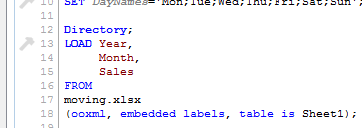Using data from attached Excel file and script from txt load data into Qlikview.

(Click on picture to see full size)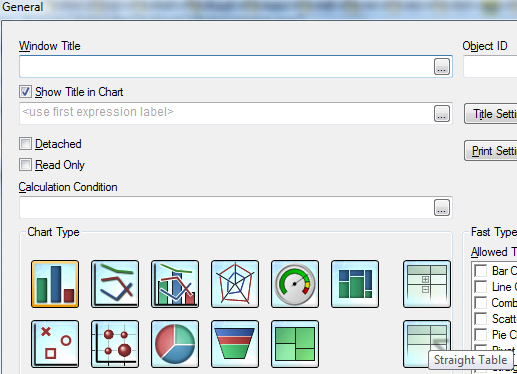Create Straight Table (Layout-->New Sheet Object-->Chart...) -->Use:

Year and Month

as dimension  -->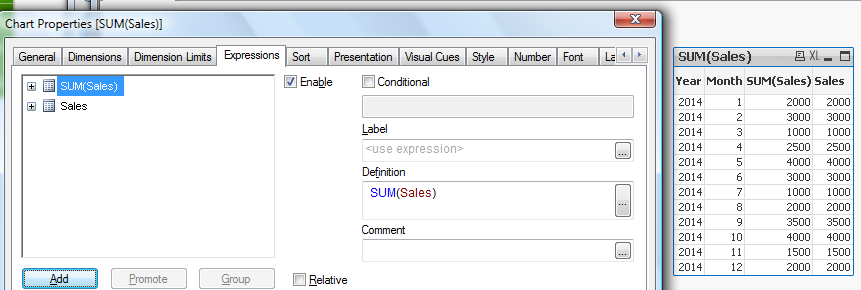and Sales as expression.

(In this example Sales and SUM(Sales) deliver the same results as we have unique values in Month dimension. -->

Investigation:

The expression we are going to take apart is:

Rangeavg(Above(Sum(Sales),1,3))

Step 1) SUM()

The syntax of SUM in Qlikview  is quite complicated when used in charts:(We not going to investigate that now so please refer to Qlikview Help for  more details)

For our example we only need:

SUM(Sales)

The result of this expression is: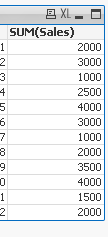Step 2) ABOVE()ABOVE takes 3 parameters:

expression - which in our example is: SUM(Sales).(we are ignoring the total here)

offset- this is integer value(0,1,2...) to specify how many rows the whole expression should be move down in relation to current row

n - this is integer value(1,2...) and will return range of values.

Remember:The last parameter will only work when used as argument with another Chart Range Function

(please see Qlikview Help for details)

The result of wrapping SUM with ABOVE

ABOVE(SUM(Sales),offset)

is  presented below.

Offset of 1,2 and 5 rows:Step 3) Rangeavg()This function returns average  of values or expressions:

Rangeavg(1,1) =1

Rangeavg(1,2,3)=2

RangeAvg(sum(1+2),sum(2+3)) is equal to RangeAvg(3,5)=4

Step 4)

So what actually is going on behind the scene we we use:

Rangeavg(Above(Sum(Sales),1,3))?

We can rephrase that expression to:

" Sum values from dimension Sales then go 1 row above and  take  values from 3 rows going up"

So based on our example :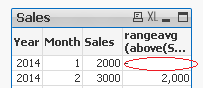Row one:

Will always return NULL

as we can not got above row 1 -->

Rangeavg()=NULL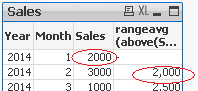Row two:

"Go up 1 row and return 3 values".

We can only go 1 row up and return 1 value

Rangeavg(2000) = 2000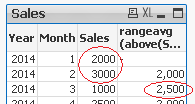Row three:

"Go up 1 and return 3 values going up"

We can only go 2 rows up:

Rangeavg (2000,3000) =

(2000+3000)/2=2500Row four:

"Go up 1 row..."

This is first row where values from 3 rows above

can be returned(Row 1, Row 2 ,Row 3)

Rangeavg(Row 1, Row 2 ,Row 3) = Rangeavg(2000+3000+1000)=6000/3=2000Row five:

Rangeavg(Row 2, Row 3 ,Row 4)

=Rangeavg(3000+1000+2500)=6500/3=2167

and so on...

The final result: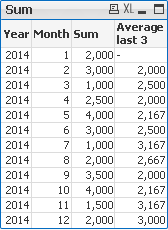Conclusions:

The offset parameter of the ABOVE function according to Qlikview Help:

"Specifying an offset greater than 1 lets you move the evaluation of expression to rows further up the current row"

I would say : greater that 0 as any values equal or greater than 1 will shift the expression down.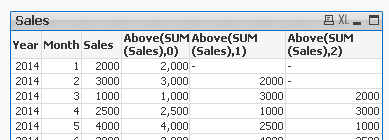In addition I would describe behaviour of the offset as:
"The offset parameter shifts the whole expression by n  (where n is integer greater than 0) rows down".

This is maybe personal way of writing but I found that easier to understand.

Case closed..?

Tags (3)
Labels (6)

• Technical BriefPartner

Hi Mika

It is great that you take the time to write these missing manuals.

I just want to add that, if you select a month in this solution, you will NOT see the average of the previous three months as you probably would expect.

This could be done using an expression like this:

Sum(

Aggr(

RangeAvg(

Above(

Sum({\$< YearMonth,Year,Month>} SalesAmount)

,1,3)

),YearMonth))

It is important though, that your is sorted correctly (required by the AGGR()).

Keep posting these missing manuals.

//MartinMaster III

Thank you Martin,

This is "case"( example) of how to solve particular problem and is not part of missing Manual series but I really appreciate your input and hope that with help other users as well.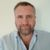Master II

I can see the use of the Above and offset function being quiet useful for bf, cf functions.

Thanks fot sharing.

Version history
Last update:
‎2015-02-24 01:41 AM
Updated by: